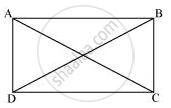# If the Diagonals of a Parallelogram Are Equal, Then Show that It is a Rectangle. - Mathematics

If the diagonals of a parallelogram are equal, then show that it is a rectangle.

#### SolutionLet ABCD be a parallelogram. To show that ABCD is a rectangle, we have to prove that one of its interior angles is 90º.

In ΔABC and ΔDCB,

AB = DC (Opposite sides of a parallelogram are equal)

BC = BC (Common)

AC = DB (Given)

∴ ΔABC ≅ ΔDCB (By SSS Congruence rule)

⇒ ∠ABC = ∠DCB

It is known that the sum of the measures of angles on the same side of transversal is 180º.

∠ABC + ∠DCB = 180º (AB || CD)

⇒ ∠ABC + ∠ABC = 180º

⇒ 2∠ABC = 180º

⇒ ∠ABC = 90º

Since ABCD is a parallelogram and one of its interior angles is 90º, ABCD is a rectangle.

Concept: Another Condition for a Quadrilateral to Be a Parallelogram
Is there an error in this question or solution?
Chapter 8: Quadrilaterals - Exercise 8.1 [Page 146]

#### APPEARS IN

NCERT Class 9 Maths